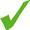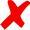# 1336 - Data Sufficiency

Data Sufficiency MCQ No. : 49512

If the units digit of integer n is greater than 2, what is the units digit of n ?

(1) The units digit of n is the same as the units digit of n2.

(2) The units digit of n is the same as the units digit of n3.### Practice Questions in Sets

All questions (MCQ) are arranged in sets of questions on pages. You will see 12 questions pre page.